# ２７　Entropic gravity／Emergent gravity　#Gravity #universe

２７　Entropic gravity／Emergent gravity　#Gravity #universe

２７－１　Outline
○Entropic gravity, also known as emergent gravity, is a theory in modern physics that describes gravity as an entropic force. 

○In 2009, Erik Verlinde disclosed a conceptual model that describes gravity as an entropic force. He argues (similar to Jacobson's result) that gravity is a consequence of the "information associated with the positions of material bodies". This model combines the thermodynamic approach to gravity with Gerard 't Hooft's holographic principle. It implies that gravity is not a fundamental interaction, but an emergent phenomenon which arises from the statistical behavior of microscopic degrees of freedom encoded on a holographic screen. 

○The theory holds that when gravity becomes vanishingly weak—levels seen only at interstellar distances—it diverges from its classically understood nature and its strength begins to decay linearly with distance from a mass. 

○From this theory it is not necessary to assume dark matter.

○The theory has been controversial within the physics community but has sparked research and experiments to test its validity. 

２７－２　Thermodynamics and the Laws of Newton
・・・Based on the paper by Erik Verlinde in 2010 

○Gravity is considerably harder to combine with quantum mechanics than all the other forces. Also string theory and its related developments have given several indications in this direction.

○The universality of gravity suggests that its emergence should be understood from
general principles that are independent of the specific details of the underlying microscopic theory.Changes in this entropy when matter is displaced leads to an entropic force, which as we will show takes the form of gravity. Its origin therefore lies in the tendency of the microscopic theory to maximize its entropy.

○The conceptual ideas behind the emergence of spacetime and gravity appear to be general and are in principle applicable to other geometries than Anti-de Sitter space：a universe closer to our own, namely de Sitter space.

○The first indication of the emergent nature of spacetime and gravity comes from the laws of black hole thermodynamics. A central role herein is played by the Bekenstein-Hawking entropy and Hawking temperature given by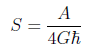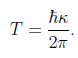Here A denotes the area of the horizon and κ equals the surface acceleration.
○ In thermodynamics of ordinary materials, entropy is proportional to volume, but in black holes it is proportional to surface area.→Holographic principle

※The formula of Bekenstein-Hawking holds in any dimension of gravity theory, G is the Newton's constant in that dimension.

○Newton's law can be obtained from the law of thermodynamics as follows.
（１）Particle and Entropy
Motivated by Bekenstein's argument, as the particles of mass m approach a screen, the entropy change ΔS on the screen becomes,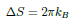where kB is Boltzman's constant.
※ Entropy ：
1) In thermodynamics (an area of physics dealing with heat), entropy is a measure of "disorder" in the physical system of matter and energy.
2) Entropy is a measure of the number of possible arrangements the atoms in a system can have. In this sense, entropy is a measure of uncertainty or randomness.

When Δx approaches the Compton wavelength,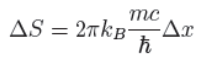The main content of this equation is simply that there is an entropy change perpendicular to the screen proportional to the mass m and the displacement Δx.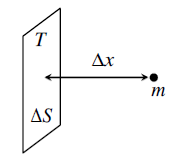Figure：A particle with mass approaches a part of the holographic screen.

※ Compton wavelength：It is a physical constant representing the mass of a particle as its length.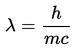※ Compton wavelength of electrons: λe = 2.42 × 10 ^-12 m

（２）Entropic force
How does force arise?
Acceleration occurs when force is applied, which is observed as temperature and entropy.

FΔx = TΔS (first law of thermodynamics)

（３）Holographic principle
Now suppose our boundary (screen) forms a closed surface. More specically, let us assume it is a sphere. The number of bits(N) is proportional to the area.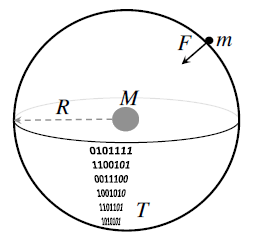Figure：A spherical holographic screen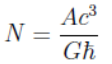A: Surface area of sphere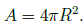c is the speed of light, ℏ is the Planck constant

※Holographic principle: The idea discovered in the field of superstring theory that information of a space can be regarded as being encoded on its boundary surface.
・For example, entropy of black hole is proportional to its surface area.
・Holographic principle is closely related to the fundamental nature of quantum theory called "quantum entanglement ".

（４）Energy and number of states (amount of information)
・Assume that there is a total energy E in this spherical holographic screen.
・Also, given the assumption that energy is equally divided into the number of states N (bits) within the boundary (law of equipartition of energy)

The energy E is proportional to the mass M when the mass M is sufficiently larger than m.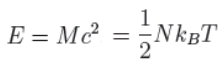T is absolute temperature.

※This fact could be true even when equipartition is not strictly obeyed.

Solving equations (1) to (4), we have recovered Newton's law of gravitation.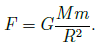○The results of this paper suggest gravity arises as an entropic force, once space and time themselves have emerged.

○The equivalence principle of acceleration and gravity are both emergent phenomena.

○Furthermore, we can obtain the Einstein equation which generalizes Newton's law. 

○ Conclusion
・It is time we nally do away with gravity as a fundamental force. If gravity is emergent, so is space time geometry. Gravity arises as an entropic force, once space and time themselves have emerged.
・For instance, the way redshifts arise from entropy gradients could lead to many new insights.

【Referendes】
1. Entropic gravity－Wikipedia
2. Erik Verlinde “On the Origin of Gravity and the Laws of Newton“ 20100106
https://arxiv.org/pdf/1001.0785.pdf
3. T. Jacobson, \Thermodynamics of space-time: The Einstein equation of state,"
Phys. Rev. Lett. 75, 1260 (1995)【Change log】
20171111　Newly posted
20171209　Addition of “ Thermodynamics and the Laws of Newton”